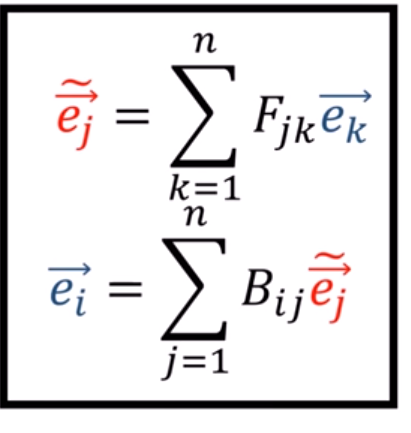# Learn Forward and backward matrix transformation for an n-dim feature space

Hi!
According to this and this, backward and forward transformations are tensor/matrices that learn move back and forth between different coordinate system. This simply means, given two vector space, it’s possible to:

1. Build a vector for the new basis from the old basis using backward (B) transformation matrix
2. Build a vector for the old basis from the new basis using Forward (F) transformation matrix

With the product of F.B = Identity matrix. also B = Inv(F)

The above images show B and F transformation matrix for 2-dimensional vector space. For n-dim vector space, we have something like thatSince the feature of an image can be thought as belonging to an n-dimensional feature space, is it possible to make pytorch learn the transformation matrices through a machine learning procedure? The two input images belong to two different views/feature space as shown below1 Like

I dont have the answer to this, new here!

But the first step of the problem would be to input the information on the neural network (better idea on what to use?)

First tough was to vectorize the matrix, but according to this paper information would be lost in the way, as well it requires much computer power, not very efficient

Matrix Neural Networks

gonna keep reading it, if I get to understand you’re going to know. The paper is about a Neural Network designed for the job